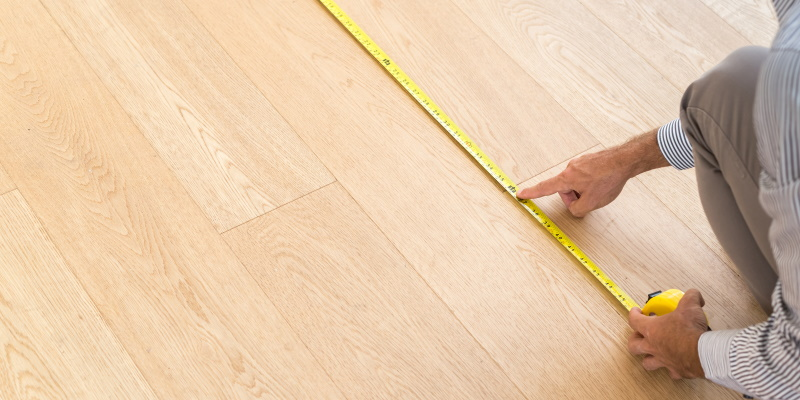# It doesn’t matter much if the tape is straightSuppose a contractor is measuring the length of a wall. He starts in one corner of the room, and lets out a tape measure heading for the other end of the wall. But something is in the way, so instead of measuring straight to the corner, he measures to a point near the corner on the adjacent wall.

If you looked down on the room from a bird’s eye view, the contractor wants to measure the distance from (0, 0) to (x, 0), but instead measures from (0, 0) to (x, y) where y is small relative to x. How much difference does this make?

The measurement error, as a function of y, is given by

(x² + y²)1/2x.

Expanding this function in a Taylor series around y = 0 shows that the error is approximately

y²/2x.

So the error is not on the order of y but of y²/x. The latter is much smaller if y is small relative to x.

For example, suppose a room is 10 feet (120 inches) long. If someone were to measure the length of the room by running a tape measure to a point 4 inches up the joining wall, the measurement error would not be anywhere near 4 inches but rather nearly 16/240 = 1/15 of an inch.

Let’s work this example out and see how good the approximation was. The hypotenuse of a right triangle with sides 120 and 4 is

√14416 = 120.066648…

which is very close to

120 + 1/15 = 120.06666…

The fact that the measurement wasn’t exactly corner-to-corner would likely not be the largest source of measurement error.